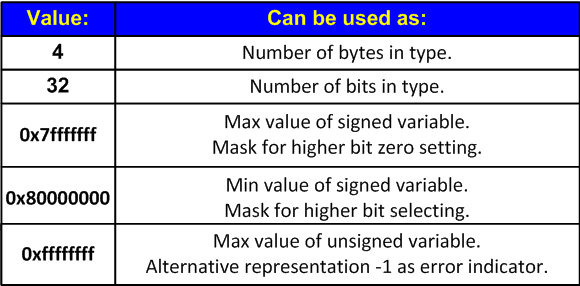﻿ V112. Dangerous magic number N used.# V112. Dangerous magic number N used.

The analyzer found the use of a dangerous magic number. The possible error may consist in the use of numeric literal as special values or size of memsize type.

Let's examine the first example.

``````size_t ArraySize = N * 4;
size_t *Array = (size_t *)malloc(ArraySize);``````

A programmer while writing the program relied on that the size 'size_t' will be always equal 4 and wrote the calculation of the array size "N * 4". This code does not take into account that 'size_t' on the 64-bit system will have 8 bytes and will allocate less memory than it is necessary. The correction of the code consists in the use of 'sizeof' operator instead of a constant 4.

``````size_t ArraySize = N * sizeof(size_t);
size_t *Array = (size_t *)malloc(ArraySize);``````

The second example.

``````size_t n = static_cast<size_t>(-1);
if (n == 0xffffffffu) { ... }``````

Sometimes as an error code or other special marker the value "-1" is used which is written as "0xffffffff". On the 64-bit platform the written expression is incorrect and one should evidently use the value "-1".

``````size_t n = static_cast<size_t>(-1);
if (n == static_cast<size_t>(-1)) { ... }``````

Let's list magic numbers which may influence the efficiency of an application while porting it on the 64-bit system and due to this are diagnosed by analyzer.You should study the code thoroughly in order to see if there are magic constants and replace them with safe constants and expressions. For this purpose you may use 'sizeof()' operator, special value from <limits.h>, <inttypes.h> etc.

In some cases magic constants are not considered unsafe. For example, there will be no warning on this code:

``float Color;``

411
14 100

### Do you make errors in the code?